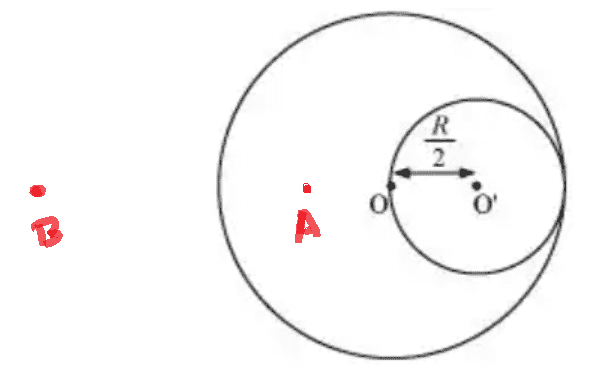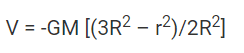# Gravitational Field and Potential at certain point

• songoku
In summary, the problem involves finding the gravitational field at point A, which is inside a larger sphere and outside a smaller one. The removed mass is ##\frac{1}{8}M## and the relevant equations are ##g=\frac{GM}{R^3}r##, ##g=\frac{GM}{r^2}##, and ##V=-\frac{GM}{r}##. The correct formula for the potential at point A is ##V=-\frac{GM(3R^2-r^2)}{2R^3}-\left(-\frac{G(\frac{1}{8}M)}{R}\right)##.

#### songoku

Homework Statement
A planet of mass ##M## has a part of it removed as shown in the diagram. Find the gravitational field at point A (which is 1/2 R from O) and point B (which is 2R from surface)
Relevant Equations
##g=\frac{GM}{r^2}##

##g=\frac{GM}{R^3}r##

##V=-\frac{GM}{r}##The removed mass is ##\frac{1}{8}M##

My idea is to find ##g## from large sphere then minus it with ##g## from small sphere (because of the removed mass):

##g## at A =
$$\frac{GM}{R^3}\left(\frac{1}{2}R\right)-\frac{G\left(\frac{1}{8}M\right)}{R^2}$$

Is this correct? Thanks

#### Attachments

•bob012345
songoku said:
Homework Statement:: A planet of mass ##M## has a part of it removed as shown in the diagram. Find the gravitational field at point A (which is 1/2 R from O) and point B (which is 2R from surface)
Relevant Equations:: ##g=\frac{GM}{r^2}##

##g=\frac{GM}{R^3}r##

##V=-\frac{GM}{r}##

View attachment 296979
The removed mass is ##\frac{1}{8}M##

My idea is to find ##g## from large sphere then minus it with ##g## from small sphere (because of the removed mass):

##g## at A =
$$\frac{GM}{R^3}\left(\frac{1}{2}R\right)-\frac{G\left(\frac{1}{8}M\right)}{R^2}$$

Is this correct? Thanks
Looks right.

•songoku
Just remember the gravitational field is a vector.

•songoku
When calculating potential gravity at point D due to large sphere, is it correct to use R as the distance since the potential gravity inside the sphere is constant and the same as at the surface?

Thanks

•songoku
songoku said:
When calculating potential gravity at point D due to large sphere, is it correct to use R as the distance since the potential gravity inside the sphere is constant and the same as at the surface?

Thanks
Where is point D?

•songoku
songoku said:
When calculating potential gravity at point D due to large sphere, is it correct to use R as the distance since the potential gravity inside the sphere is constant and the same as at the surface?

Thanks
Not sure what you are asking here. Is this a new problem or did you mean point ##B##? Please draw it. Thanks.

•songoku
kuruman said:
kuruman said:
Where is point D?
bob012345 said:
Not sure what you are asking here. Is this a new problem or did you mean point ##B##? Please draw it. Thanks.
I am really sorry, I mean point A (got mixed up with other question I am doing)

Thanks

songoku said:
I am really sorry, I mean point A (got mixed up with other question I am doing)

Thanks
since the potential gravity inside the sphere is constant and the same as at the surface?

Still not sure what you mean here. You did point ##A## correctly in the first post.

•songoku
bob012345 said:
since the potential gravity inside the sphere is constant and the same as at the surface?

Still not sure what you mean here. You did point ##A## correctly in the first post.
This is how I calculate gravitational potential at point A:

V at A =
$$-\frac{GM}{R}-\left(-\frac{G\left(\frac{1}{8}M\right)}{R}\right)$$

Is this correct? Thanks

songoku said:
This is how I calculate gravitational potential at point A:

V at A =
$$-\frac{GM}{R}-\left(-\frac{G\left(\frac{1}{8}M\right)}{R}\right)$$

Is this correct? Thanks
Sorry, that does not look correct.

•songoku
songoku said:
When calculating potential gravity at point D due to large sphere, is it correct to use R as the distance since the potential gravity inside the sphere is constant and the same as at the surface?

Thanks
No. This is only possible inside a spherical shell.

•songoku
bob012345 said:
Sorry, that does not look correct.
Orodruin said:
No. This is only possible inside a spherical shell.Is that the formual I need to use? So maybe like this: V at A =

$$V=-\frac{GM(3R^2-(\frac{1}{4}R^2)}{2R^2}-\left(-\frac{G(\frac{1}{8}M}{R}\right)$$

Thanks

This is not dimensionally correct. How did you get it? Maybe your method is correct but not the execution. We can't tell unless you show us what you did.

•songoku
kuruman said:
This is not dimensionally correct. How did you get it? Maybe your method is correct but not the execution. We can't tell unless you show us what you did.
I googled the formula for potential inside solid sphere but I got the wrong formula. This is the correct one:
$$V=-\frac{GM(3R^2-r^2)}{2R^3}$$

So V at A should be:
$$V=-\frac{GM(3R^2-\frac{1}{4}R^2)}{2R^3}-\left(-\frac{G(\frac{1}{8}M)}{R}\right)$$

Is that correct? Thanks

How do you know that this formula that you googled is correct? In post #4
songoku said:
When calculating potential gravity at point D due to large sphere, is it correct to use R as the distance since the potential gravity inside the sphere is constant and the same as at the surface?
you seem to think that the potential inside the sphere is constant and the same as at the surface. The formula you found says that the potential is not constant inside and that it is equal to the potential at the surface only at the center. Which is correct and why?

I think that the intent here is for you to do the derivation yourself, not google it. Then you will have learned something other than "I can always google the answer." The googled answer should be a check whether you did it right.

The problem is asking you to find the gravitational field at a point inside the larger sphere and outside the smaller one (point A) and also outside both spheres at point B which you haven't done so far. Knowing the expressions for the fields, the idea then is to find the potential at points inside and outside by doing the appropriate line integrals.

•songoku
kuruman said:
How do you know that this formula that you googled is correct?
I don't, I just compare several links to check

kuruman said:
you seem to think that the potential inside the sphere is constant and the same as at the surface. The formula you found says that the potential is not constant inside and that it is equal to the potential at the surface only at the center. Which is correct and why?
Orodruin told me in post#12 that potential inside the sphere is constant and the same as at the surface is only for spherical shell so I tried to find formula for solid sphere. The correct one is ##V=-\frac{GM(3R^2-r^2)}{2R^3}## because the question is about solid sphere, not shell.

kuruman said:
I think that the intent here is for you to do the derivation yourself, not google it. Then you will have learned something other than "I can always google the answer." The googled answer should be a check whether you did it right.
I am not sure whether the formula derivation is part of the lesson / curriculum because the students have never been asked to derive any formulas since the beginning of the class. I know the derivation but only because I looked it up, not because I did it myself.

kuruman said:
The problem is asking you to find the gravitational field at a point inside the larger sphere and outside the smaller one (point A) and also outside both spheres at point B which you haven't done so far. Knowing the expressions for the fields, the idea then is to find the potential at points inside and outside by doing the appropriate line integrals.
##g## at B:
$$g_B=\frac{GM}{9R^2}-\frac{G(\frac{1}{8}M)}{\frac{49}{4}R^2}=\frac{89}{882}\frac{GM}{R^2}$$

V at A:
$$V_A=-\frac{GM(3R^2-\frac{1}{4}R^2)}{2R^3}-\left(-\frac{G(\frac{1}{8}M)}{R}\right)$$

V at B:
$$V_B=-\frac{GM}{3R}-\left(-\frac{G(\frac{1}{8}M)}{\frac{7}{2}R}\right)$$

Is the idea correct? Thanks

songoku said:
I don't, I just compare several links to check

Orodruin told me in post#12 that potential inside the sphere is constant and the same as at the surface is only for spherical shell so I tried to find formula for solid sphere. The correct one is ##V=-\frac{GM(3R^2-r^2)}{2R^3}## because the question is about solid sphere, not shell.

I am not sure whether the formula derivation is part of the lesson / curriculum because the students have never been asked to derive any formulas since the beginning of the class. I know the derivation but only because I looked it up, not because I did it myself.

##g## at B:
$$g_B=\frac{GM}{9R^2}-\frac{G(\frac{1}{8}M)}{\frac{49}{4}R^2}=\frac{89}{882}\frac{GM}{R^2}$$

V at A:
$$V_A=-\frac{GM(3R^2-\frac{1}{4}R^2)}{2R^3}-\left(-\frac{G(\frac{1}{8}M)}{R}\right)$$

V at B:
$$V_B=-\frac{GM}{3R}-\left(-\frac{G(\frac{1}{8}M)}{\frac{7}{2}R}\right)$$

Is the idea correct? Thanks
Without doing the math myself it seems all right but you can check it by deriving the field from the potential and see if they match.

•songoku
bob012345 said:
Without doing the math myself it seems all right but you can check it by deriving the field from the potential and see if they match.
##g## at A =
$$\frac{GM}{R^3}\left(\frac{1}{2}R\right)-\frac{G\left(\frac{1}{8}M\right)}{R^2}=\frac{3}{8}\frac{GM}{R^2}$$

V at A:
$$V_A=-\frac{GM(3R^2-\frac{1}{4}R^2)}{2R^3}-\left(-\frac{G(\frac{1}{8}M)}{R}\right)=-\frac{5}{4}\frac{GM}{R}$$

Then:
$$-\frac{dV_A}{dR}=\frac{5}{4}\frac{GM}{R^2}$$

which is not the same as ##g_A##, but I can't find where I went wrong. Where is my mistake? Thanks

songoku said:
Where is my mistake?
Differentiating wrt R is not going to give the field at A. You want how the potential changes if you move a little from A, but R is not a measure of displacement from A. If you change R the whole setup changes.

•songoku
haruspex said:
Differentiating wrt R is not going to give the field at A. You want how the potential changes if you move a little from A, but R is not a measure of displacement from A. If you change R the whole setup changes.
I understand. I am just crazy to take derivative wrt R because R is constant.

Thank you very much for all the help and explanation haruspex, bob012345, kuruman, Orodruin

•bob012345